Next: Hierarchical modelling and hyperparameters Up: Inferring numerical values of Previous: Multidimensional case

## Predictive distributions

A related problem is to infer' what an experiment will observe given our best knowledge of the underlying theory and its parameters. Infer is within quote marks because the term is usually used for model and parameters, rather than for observations. In this case people prefer to speak about prediction (or prevision). But we recall that in the Bayesian reasoning there is conceptual symmetry between the uncertain quantities which enter the problem. Probability density functions describing not yet observed event are referred to as predictive distributions. There is a conceptual difference with the likelihood, which also gives a probability of observation, but under different hypotheses, as the following example clarifies.

Given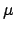and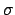, and assuming a Gaussian model, our uncertainty about a future'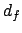is described by the Gaussian pdf Eq. (25) with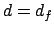. But this holds only under that particular hypothesis forand, while, in general, we are also uncertain about these values too. Applying the decomposition formula (Tab. 1) we get: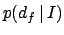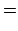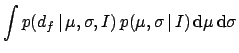(58)

Again, the integral might be technically difficult, but the solution is conceptually simple. Note that, though the decomposition formula is a general result of probability theory, it can be applied to this problem only in the subjective approach.

An analytically easy, insightful case is that of experiments with well-known's. Given a past observation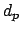and a vague prior,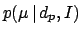is Gaussian aroundwith variance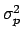[note that, with respect to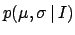of Eq.(58), it has been made explicit thatdepend on].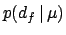is Gaussian aroundwith variance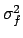. We get finally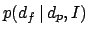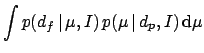(59)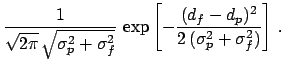(60)Next: Hierarchical modelling and hyperparameters Up: Inferring numerical values of Previous: Multidimensional case
Giulio D'Agostini 2003-05-13